International Journal of Intelligent Information Systems
Volume 5, Issue 5, October 2016, Pages: 71-74

Phase Matching Denoising Algorithm and IP Design

Wu Peng, Xia Hui, Wang Chen

ShenYang Normal University Software College, ShenYang, China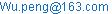(Wu Peng)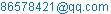(Xia Hui)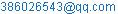(Wang Chen)

Wu Peng, Xia Hui, Wang Chen. Phase Matching Denoising Algorithm and IP Design. International Journal of Intelligent Information Systems. Vol. 5, No. 5, 2016, pp. 71-74. doi: 10.11648/j.ijiis.20160505.13

Received: September 18, 2016; Accepted: October 10, 2016; Published: October 17, 2016

Abstract: At present, there are many kinds of denoising algorithms, but the hardware of these algorithms IP design is very lacked. In this paper, a phase matching denoising algorithm is proposed to remove the noise, and the IP design of this method is realized. Phase matching denoising algorithm is based on the signal phase matching method to obtain the actual signal and remove noise. Mathematical expression and mathematical proof of the method are given here. Using this method, the IP kernel design is given and the design method is given. Through the function simulation, it is proved that the IP kernel design can effectively remove the noise in the signal.

Keywords: Phase Match, IP Kernel, De-noise, Simulation

Contents

1. Introduction

With the continuous development of science and technology, the current electronic system is a powerful and complex structure. Because of the complex system structure, the system development is generally divided into several levels . Each level is composed of a number of modules. In the actual development process, the soft IP and hard IP can be used as the development process components. They can be placed in a resource pool when the system needs to be called. Signal denoising IP kernel is very lacked . In addition, design method based on an integrated platform is a kind of stable IP framework design method. Based on these reasons , Quartus Prime design platform is used to design IP kernel of the phase matching denoising algorithm.

2. Phase Matching Denoising Algorithm

Phase matching denosing algorithm uses two sensor line array to receive signal. The received signal is represented by a formula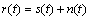, the received signal is the original signal and noise signal linear additivity.  Figure 1 shows a linear array of two elements, the array element 1 and the array element 2 simultaneously receive the signal .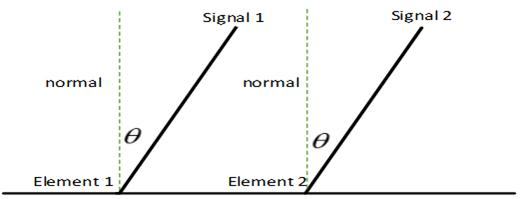Fig. 1. Signal receiving array.

In Figure 1 the array element 1 receives signal 1 and element 2 receives signal 2. Mathematical equations is expressed as the following form:q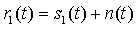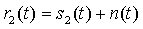(1)

In equations (1)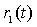and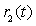are the signal received by the array element. They are known signal.and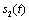are the original signal which need to restore and desired signal.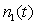and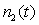are isotropic random noise.

In the noise environment, the distance between array element 1 and the array element 2 is close and the signal acquisition is at the same time [5, 6]. The received noise is close to the same noise because of the isotropic noise. the equations (1) can be written in the following form: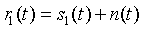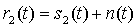(2)

Fourier transform of the two sides of the equations (2) can be obtained as follows: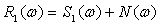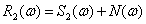(3)

In order to study the problem, the equations (3) is expressed as follows in the frequency domain polar coordinates: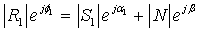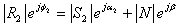(4)

In equations (4),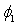,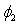,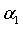,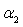and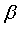are respectively the phase angle of the received signal, the the desired signal and the noise signal.These phase angles are the functions of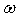.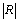,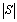and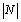are respectively the spectrum amplitude of the received signal, the desired signal and the noise signal. Phase angle is the antitangent value of ratio. The ratio is the ratio of the real part and the imaginary part of the Fourier transform.

The distance between the array element 1 and the element 2 is set to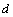. The included angle between the original signal and the normal of the two element array is set to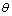. For far field signals, the arrival time of the wave front is not the same. Time difference is expressed as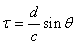,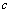is the wave velocity[7,8]. Because of the arrival time difference, The two original signal phase is different between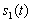and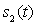.Desired signal phase isaligned in the complex domain. Desired signal phase alignment is expressed as follows: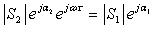(5)

Take (5) to (4), gets the following equations: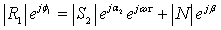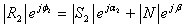(6)is obtained by the equation group (6),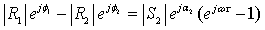(7)

The equation (7) plural frequency domain transform  is expressed as follwos: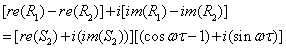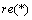is the real part of the plural.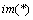is the imaginary part of the plural.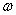is the angular frequency of the signal. If two complex numbers are equal, the real and imaginary parts of the two complex numbers are equal. The following equations are obtained: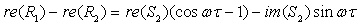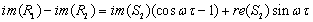Equations solution is abtained as follows: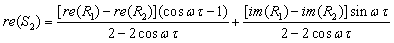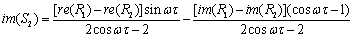The real part of the desired signal is calculated by the real part and imaginary part of received signals by Fourier transform. The imaginary part of the desired signal can also be alculated. After the desired signal is calculated in the frequency domain, it can estimate the expected time domain waveform of the original signal by the inverse Fourier transform.The phase matching method can resist and eliminate the noise. Desired signal can also be calculated. For low signal to noise ratio signal, do not need to know the type of noise.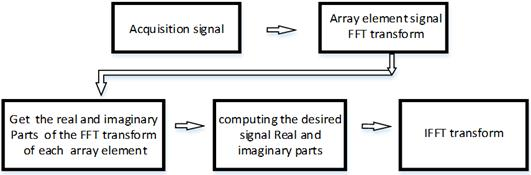Fig. 2. Phased matching denoising algorithm steps.

Figure 2 shows the phase matching denoising algorithm steps. The first is the acquisition of the signal at the same time in the array. Secondly, the signal is collected by Fourier transform, and the signal phase is aligned. Finally, the real and imaginary parts of the desired signal are calculated by the real and imaginary parts of the received signal, and then the desired signal is obtained through the inverse Fourier transform.

3. Phase Matching Denoising Algorithm IP Kernel

According to the content of the phase matching denoising algorithm, the IP kernel design is divided into four parts: input, processing, output and control. The input part is the process of the signal sampling, and the sampling data is stored in the memory. Processing part is that the sampling signal in memory is read out and transformed by Fourier transform. By sampling signal real and imaginary parts, the real and imaginary parts of the desired signal are calculated, and the inverse Fourier transform is carried out to restore the desired signal in time domain. The output part is to output the processing result. In fact, the output signal is stored in the output memory. Control part is to coordinate the other parts to work orderly through the control signal. In the structure of the IP kernel design, the part that needs special explanation is the control part. This part is the key of the IP kernel design. This part controls the whole data flow and coordinate each part to work.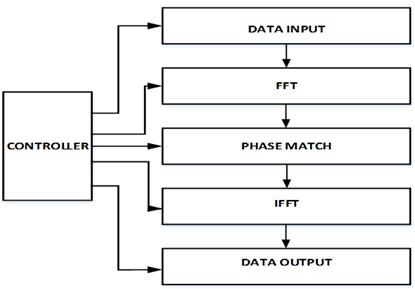Fig. 3. IP kernel integral structure.

The overall structure of IP kernel is designed as six modules which are control module, data input module, fast Fourier transform module, phase matching module, inverse Fourier transform module and data output module.

The phase matching module will match the data after Fourier transform, the structure of the model is shown in Figure 4.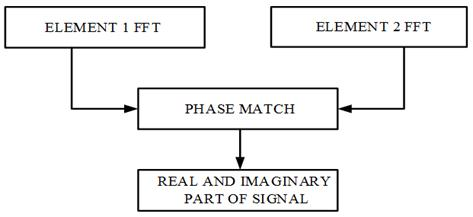Fig. 4. Integral structure on phased match.

The signal received by the array element 1 and the array element 2 is done by Fourier transform. The transformed results are given to the phase matching part. The matching operation is carried out to obtain the real part and the imaginary part of the desired signal.

4. Simulation

Phase matching denoising algorithm is to receive signals from the sensor. After Fourier transform and phase matching, get rid of noise to get the desired signal. The structure of the IP kernel can be divided into three parts, the Fourier transform part, the phase matching part and the inverse Fourier transform. The several parts can be worked in a pipeline manner. Each part can be run independently and is the output and input relation. The parallel operation can be formed. Control module gives the control signal, so that each part is work oderly[10,11].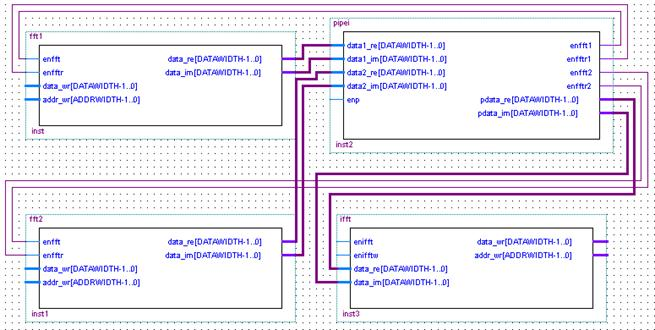Fig. 5. Double array phase match algorithm schematic.

In Fig. 5 the pipei section receives signals real part and imaginary part from fft1 and fft2. The phase matching operation is carried out under the control signal enp, and the result of the operation is output to the ifft part. enifft signal control ifft part which is the inverse Fourier transform. Under the control of enifftw signal, time domain data is output.

The original signal is a sine wave of 100Hz frequency. The Gauss white noise is added to the sine wave signal. The signal to noise ratio is -1dB. The number of sampling point is 10K.The IP kernel function simulation of denoising effect is shown in figure6.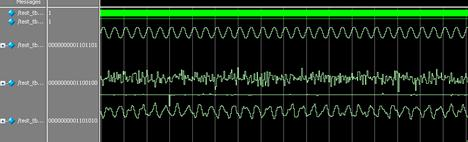Fig. 6. Phase match denoising algorithm function simulation.

The upper part of the Fig. 6 is the original sine signal. The central part of the figure is the sine signal added Gauss noise. The signal has become chaotic, has not distinguish what is the signal. The lower part of the Fig. 6 is the recovery signal after phase matching. The recovered signal can be seen as a sinusoidal signal.

5. Conclusion

In this paper, the phase matching denoising algorithm and the IP kernel design method are given. The algorithm is analyzed and proved. The IP kernel module design is given. The phase matching part realization of IP kernel is described. Simulation results show that the phase matching denoising algorithm IP kernel design is correct. The IP kernel can remove the Gauss white noise in the low signal to noise ratio, which provides the design idea for the denoising IP kernel design.

References

1. Wu SongLing. The development and Prospect of IP kernel technology in space system[J].Aerospace Standardization,2012,(2):1-3.
2. Cui XuJing,Ma PingQuan. Design and Implementation of Touch Screen Controller IP Kernel based on SOPC[J].Electronic Design Engineering, 2012,20(14):166-169.
3. Liu WenChao,Huang HanHua,Tang WenLong. Design and Implementation of Infrared Decoder IP core based on SoPC[J]. Modern Electronics Technique, 2012, 35(12):4-6.
4. Wu Peng,LI Jing-jiao,Wang Ai-xia,Wang Jiao.Higher Order Spectra Denoising Method ofPhase Matching Noise Estimation,[J].Journal of Chinese Computer System,2010,31(12):2381-2384.
5. Wu Peng, LiuJie, LiJingjiao. Wavelet Denoising of the Noise Estimation on Phase Matching, [J]. International Journalof Innovative Computing Information and Control, 2011, 7(6): 3073-3081.
6. Liu Li,Zhu Weijie, Sun Jincai.Method of Tatal Least Squares of Parameter Estimation for Sinusoidal Signals Using Principle of Signal Matched-phase, [J].Acoustic technique, 2004, 23(1): 128-132.
7. Niu Yi-long, Xiao Hui,Sun Jin-cai,YangHong-hui. A Genetic Algorithm for DOA and arameter Estimation based on Signal Phase Matching Principle, [J]. Journal of Harbin institute of technology, 2008, 40(7): 156-160.
8. WuPeng, LiJingJiao.The Noise Removal onRebound Signal of Linear Fequency[C],Proceedings 2009 9th internatianal conference on hybrid intelligent systems HIS 2009,3:7-9.
9. Soo Changpe, Jian Jiunding. Relations between Fractional Operationsand Time-frequencyDistributions, and their applications. IEEE Transon SP, 2001,49(8):1638-1655.
10. Wu ZhengGuo,XiaLi,Yin WeiMin. Modern Signal Processing Technology[M].Wuhan University press,2003,15-75.
11. KangLei,Song CaiLi,Li RuiZhou. Digital Circuit Design and Implementation ofHDL Verilog[M]. Xi'an Electronic and Science University press,2010,385-395.

 Contents 1. 2. 3. 4. 5.
Article ToolsAbstractPDF(320K)